# ESP32 - Ultrasonic Sensor

In this tutorial, we are going to learn:

• How to ultrasonic sensor works
• How to use ultrasonic sensor HC-SR04 to measure the distance using ESP32
• How to filter noise from distance measurements of ultrasonic sensor

## Hardware Required

 1 × ESP-WROOM-32 Dev Module 1 × Micro USB Cable 1 × Ultrasonic Sensor 4 × Jumper Wires
Please note: These are affiliate links. If you buy the components through these links, We may get a commission at no extra cost to you. We appreciate it.

Ultrasonic sensor HC-SR04 is used to measure the distance to an object by using ultrasonic waves.

### Pinout

The ultrasonic sensor HC-SR04 includes four pins:

• VCC pin needs to be connected to VCC (5V)
• GND pin needs to be connected to GND (0V)
• TRIG pin this pin receives the control signal (pulse) from ESP32.
• ECHO pin this pin sends a signal (pulse) to ESP32. ESP32 measures the duration of pulse to calculate distance.
•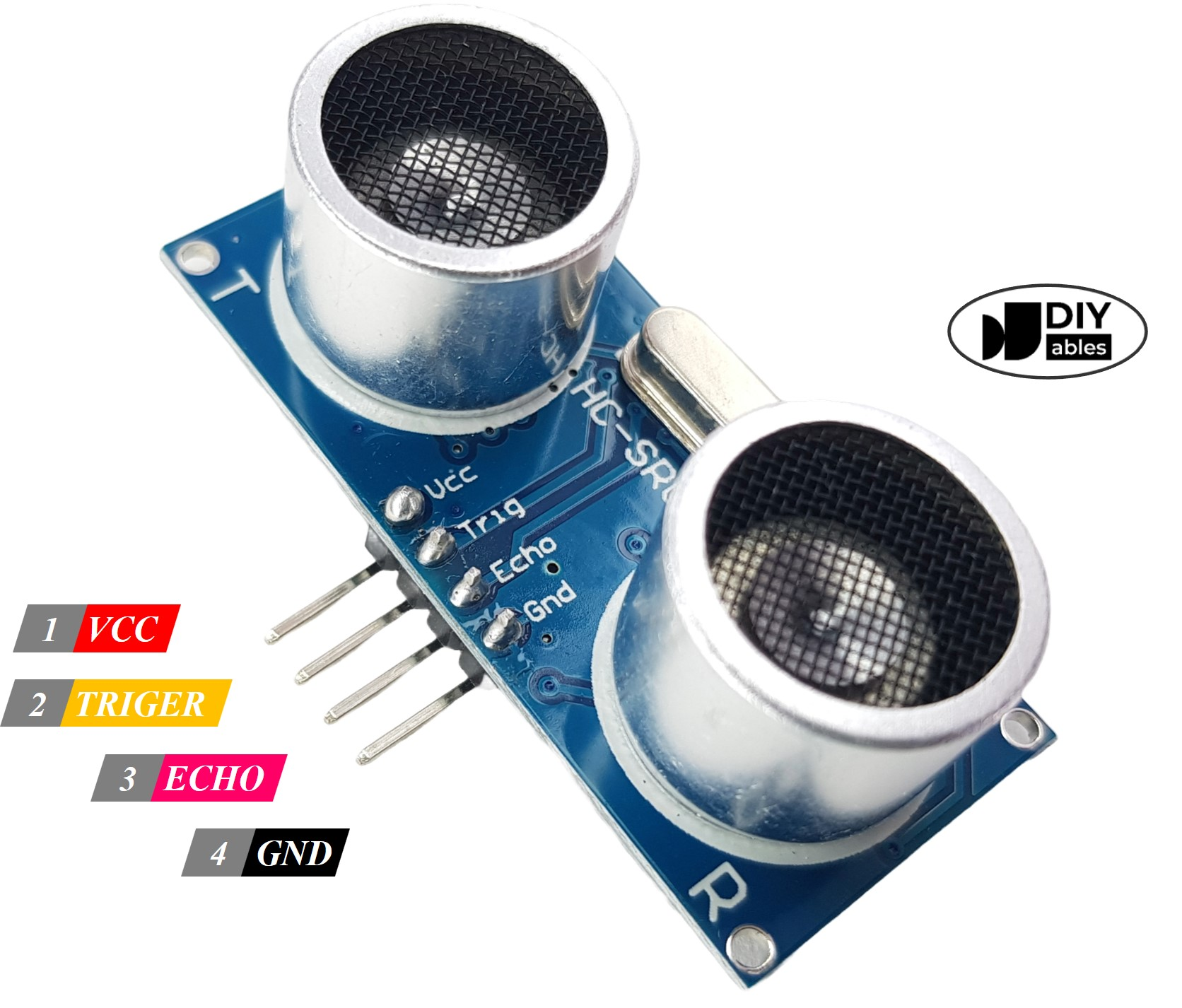### How It Works

This section is the in-depth knowledge. DON'T worry if you don't understand. Ignore this section if it overloads you, and come back in another day. Keep reading the next sections.
1. Micro-controller: generates a 10-microsecond pulse on the TRIG pin.
2. The ultrasonic sensor automatically emits the ultrasonic waves.
3. The ultrasonic wave is reflected after hitting an obstacle.
4. The ultrasonic sensor:
• Detects the reflected ultrasonic wave.
• Measures the travel time of the ultrasonic wave.
5. Ultrasonic sensor: generates a pulse to the ECHO pin. The duration of the pulse is equal to the travel time of the ultrasonic wave.
6. Micro-controller measures the pulse duration in the ECHO pin, and then calculate the distance between sensor and obstacle.

### How to Get Distance From Ultrasonic Sensor

To get distance from the ultrasonic sensor, we only need to do two steps (1 and 6 on How It Works part)

• Generates a 10-microsecond pulse on TRIG pin
• Measures the pulse duration in ECHO pin, and then calculate the distance between sensor and obstacle

#### Distance Calculation

We have:

• The travel time of the ultrasonic wave (µs): travel_time = pulse_duration
• The speed of the ultrasonic wave: speed = SPEED_OF_SOUND = 340 m/s = 0.034 cm/µs

So:

• The travel distance of the ultrasonic wave (cm): travel_distance = speed × travel_time = 0.034 × pulse_duration
• The distance between sensor and obstacle (cm): distance = travel_distance / 2 = 0.034 × pulse_duration / 2 = 0.017 × pulse_duration

### ESP32 - Ultrasonic Sensor

ESP32's pins can generate a 10-microsecond pulse and measure the pulse duration. Therefore, we can get the distance from the ultrasonic sensor by using two ESP32's pins:

• One pin is connected to TRIG PIN to generate 10µs pulse to TRIG pin of the sensor
• Another pin is connected to ECHO PIN measure pulse from the sensor

## Wiring Diagram

• Wiring diagram with power supply from USB cable
•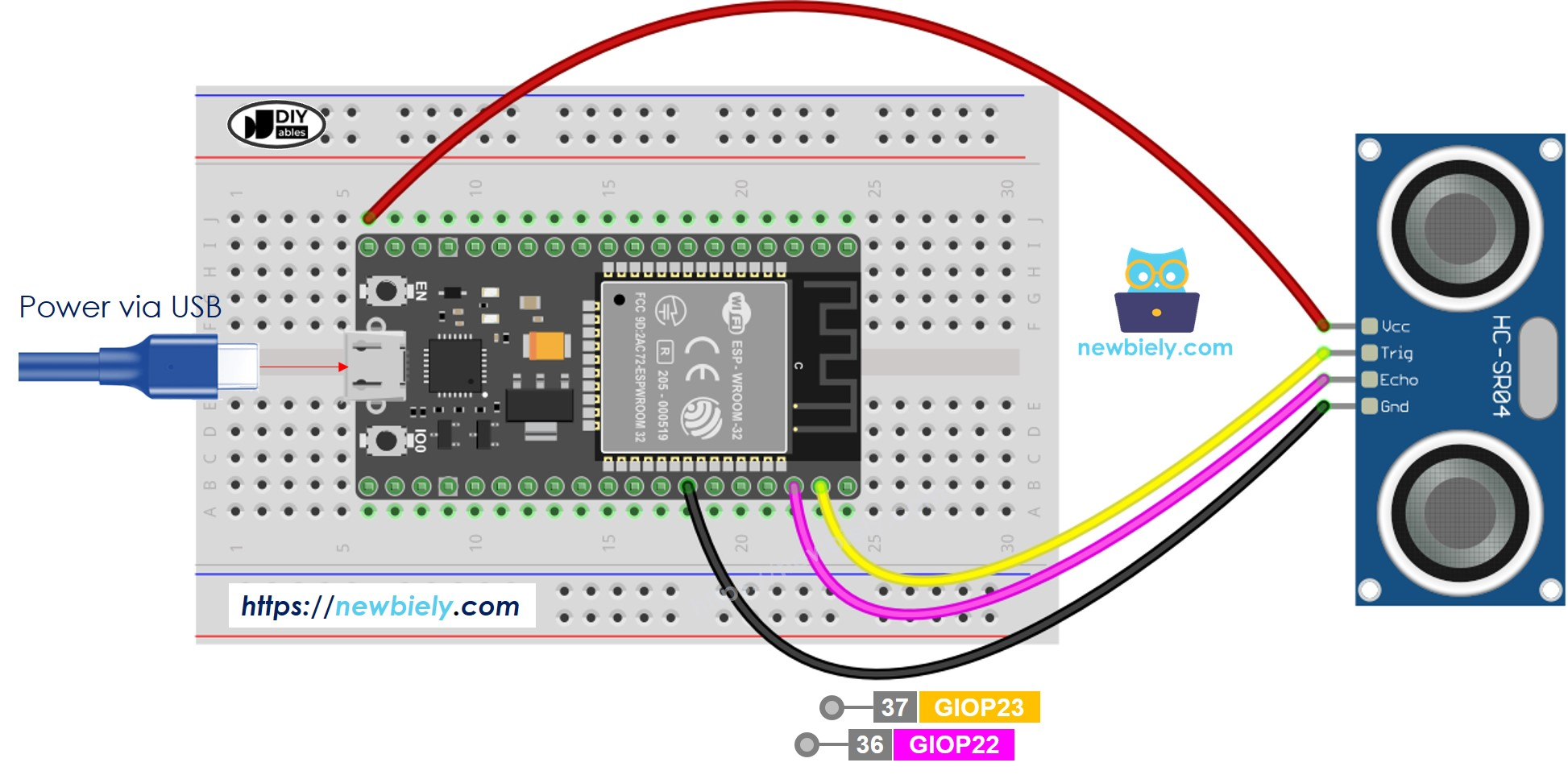Image is developed using Fritzing. Click to enlarge image

• Wiring diagram with power supply from 5v adapter
•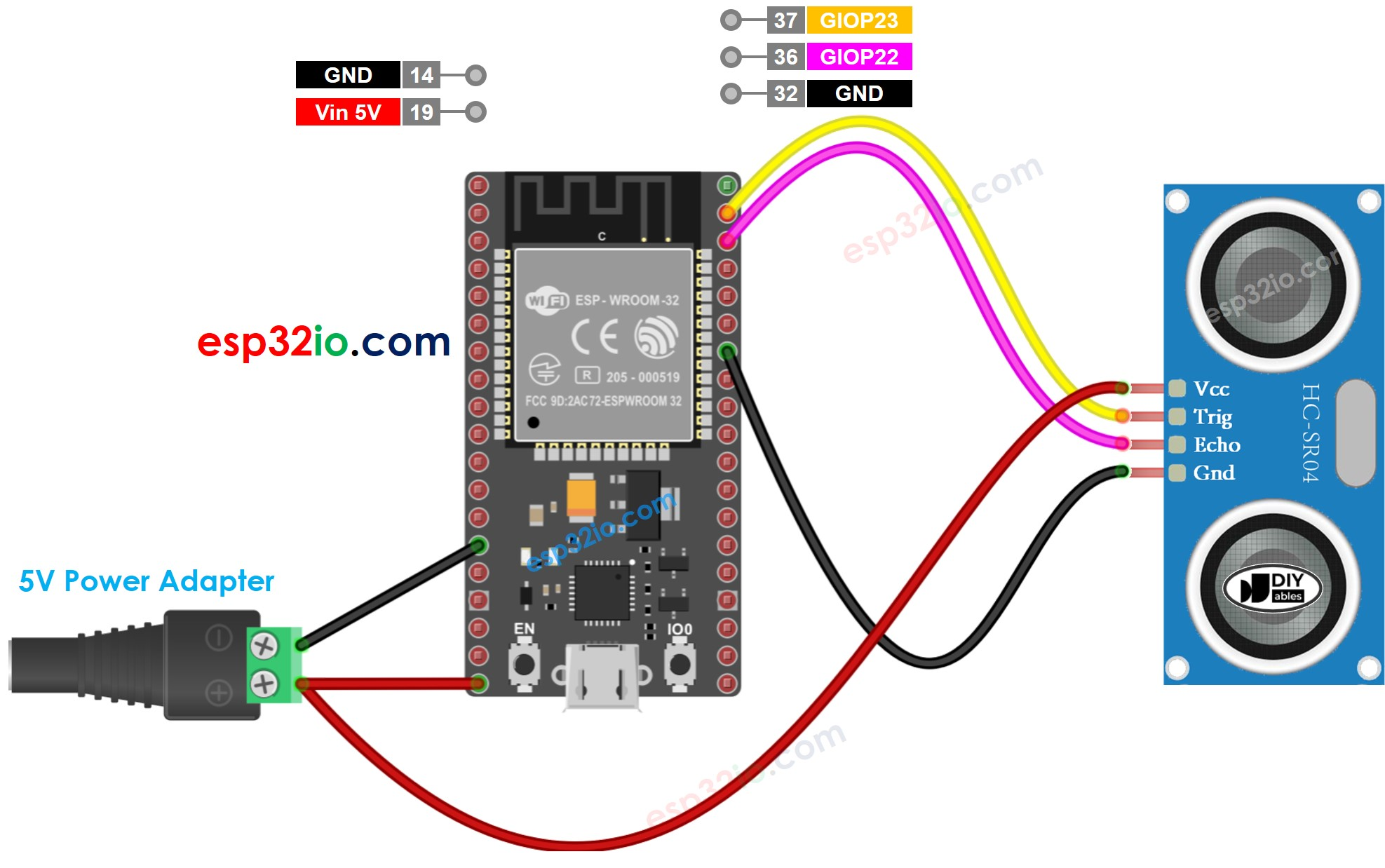Image is developed using Fritzing. Click to enlarge image

## How To Program For Ultrasonic Sensor

digitalWrite(9, HIGH); delayMicroseconds(10); digitalWrite(9, LOW);
• Measures the pulse duration (µs) in ESP32's pin by using pulseIn() function. For example, pin 8:
duration_us = pulseIn(8, HIGH);
• Calculate distance (cm):
distance_cm = 0.017 * duration_us;

## ESP32 Code

/* * Created by https://esp32io.com * * Arduino - Ultrasonic Sensor HC-SR04 * * Wiring: Ultrasonic Sensor -> Arduino: * - VCC -> 5VDC * - TRIG -> Pin GIOP23 * - ECHO -> Pin GIOP22 * - GND -> GND * * Tutorial is available here: https://esp32io.com/tutorials/arduino-ultrasonic-sensor */ const int TRIG_PIN = 23; // ESP32 pin GIOP23 connected to Ultrasonic Sensor's TRIG pin const int ECHO_PIN = 22; // ESP32 pin GIOP22 connected to Ultrasonic Sensor's ECHO pin float duration_us, distance_cm; void setup() { // begin serial port Serial.begin (9600); // configure the trigger pin to output mode pinMode(TRIG_PIN, OUTPUT); // configure the echo pin to input mode pinMode(ECHO_PIN, INPUT); } void loop() { // generate 10-microsecond pulse to TRIG pin digitalWrite(TRIG_PIN, HIGH); delayMicroseconds(10); digitalWrite(TRIG_PIN, LOW); // measure duration of pulse from ECHO pin duration_us = pulseIn(ECHO_PIN, HIGH); // calculate the distance distance_cm = 0.017 * duration_us; // print the value to Serial Monitor Serial.print("distance: "); Serial.print(distance_cm); Serial.println(" cm"); delay(500); }

### Quick Steps

• If this is the first time you use ESP32, see how to setup environment for ESP32 on Arduino IDE
• Copy the above code and paste it to Arduino IDE
• Click Upload button on Arduino IDE to compile and upload code to ESP32 board
•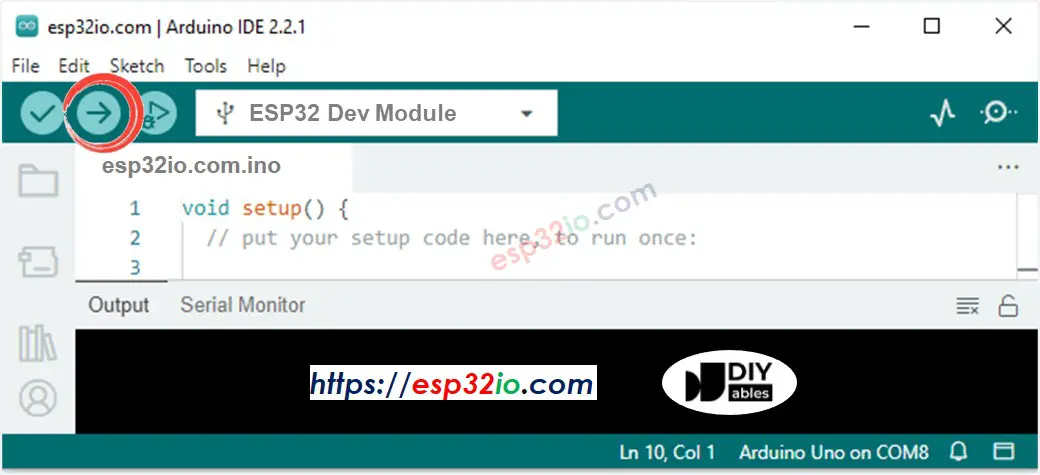• Open Serial Monitor on Arduino IDE
•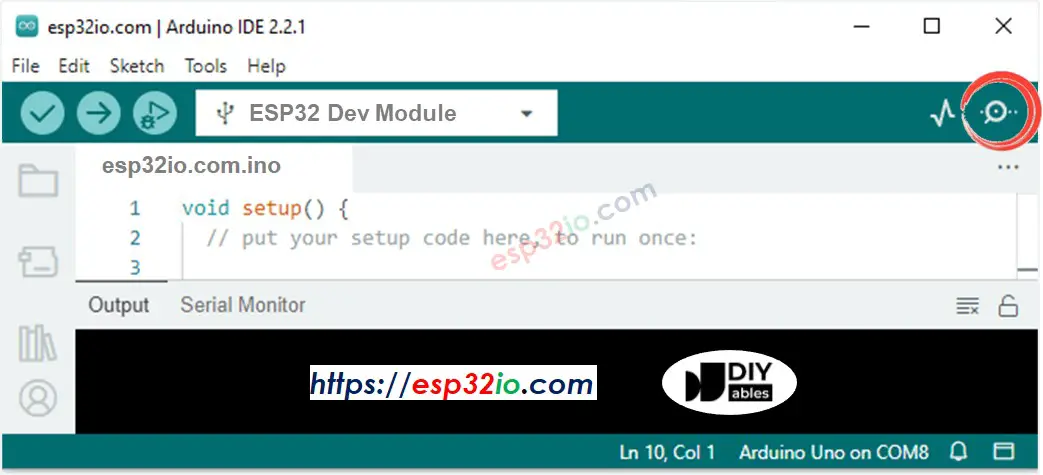• Move your hand in front of ultrasonic sensor
• See the distance from the sensor to your hand on Serial Monitor
• COM6
distance: 29.4 cm distance: 27.6 cm distance: 26.9 cm distance: 17.4 cm distance: 16.9 cm distance: 14.3 cm distance: 15.6 cm distance: 13.1 cm
Autoscroll Show timestamp
Clear output
9600 baud
Newline

### Code Explanation

Read the line-by-line explanation in comment lines of code!

## How to Filter Noise from Distance Measurements of Ultrasonic Sensor

The measurement result from ultrasonic sensor contains noise. In some application, the noised result causes the unwanted operation. We can remove noise by using the following algorithm:

1. Taking multiple measurements and store in an array
2. Sorting the array in ascending order
3. Filtering noise
• The some smallest samples are considered as noise → ignore it
• The some biggest samples are considered as noise → ignore it
• ⇒ get average of the middle samples

The below example code takes 20 measurements

• The five smallest samples are considered as noise → ignore it
• The five biggest samples are considered as noise → ignore it
• ⇒ get average of the 10 middle samples (from 5th to 14th)
/* * Created by esp32io.com * * This example code is in the public domain * * Tutorial page: https://esp32io.com/tutorials/esp32-ultrasonic-sensor */ const int TRIG_PIN = 23; // ESP32 pin GIOP23 connected to Ultrasonic Sensor's TRIG pin const int ECHO_PIN = 22; // ESP32 pin GIOP22 connected to Ultrasonic Sensor's ECHO pin float filterArray; // array to store data samples from sensor float distance; // store the distance from sensor void setup() { // begin serial port Serial.begin (9600); // configure the trigger and echo pins to output mode pinMode(TRIG_PIN, OUTPUT); pinMode(ECHO_PIN, INPUT); } void loop() { // 1. TAKING MULTIPLE MEASUREMENTS AND STORE IN AN ARRAY for (int sample = 0; sample < 20; sample++) { filterArray[sample] = ultrasonicMeasure(); delay(30); // to avoid untrasonic interfering } // 2. SORTING THE ARRAY IN ASCENDING ORDER for (int i = 0; i < 19; i++) { for (int j = i + 1; j < 20; j++) { if (filterArray[i] > filterArray[j]) { float swap = filterArray[i]; filterArray[i] = filterArray[j]; filterArray[j] = swap; } } } // 3. FILTERING NOISE // + the five smallest samples are considered as noise -> ignore it // + the five biggest samples are considered as noise -> ignore it // ---------------------------------------------------------------- // => get average of the 10 middle samples (from 5th to 14th) double sum = 0; for (int sample = 5; sample < 15; sample++) { sum += filterArray[sample]; } distance = sum / 10; // print the value to Serial Monitor Serial.print("distance: "); Serial.print(distance); Serial.println(" cm"); } float ultrasonicMeasure() { // generate 10-microsecond pulse to TRIG pin digitalWrite(TRIG_PIN, HIGH); delayMicroseconds(10); digitalWrite(TRIG_PIN, LOW); // measure duration of pulse from ECHO pin float duration_us = pulseIn(ECHO_PIN, HIGH); // calculate the distance float distance_cm = 0.017 * duration_us; return distance_cm; }

## Video Tutorial

We are considering to make the video tutorials. If you think the video tutorials are essential, please subscribe to our YouTube channel to give us motivation for making the videos.

## Challenge Yourself

Use ultrasonic sensor to do one of the following projects:

• Collision avoidance for RC car.
• Detecting the fullness of dustbin.
• Monitoring level of the dustbin.
• Automatically open/close the dustbin. Hint: Refer to ESP32 - Servo Motor.

Some manufacturers provide the ultrasonic sensor that has 3 pins. TRIG signal and ECHO signal are in the same pin. In this case, we need to use only one ESP32's pin for both purposes: generating a pulse to the sensor and measuring pulse from the sensor.

## Alternative Ultrasonic Sensors

The above code also works with the following ultrasonic sensor:

## Ultrasonic Sensor Applications

• Collision Avoidance
• Fullness Detection
• Level Measurement
• Proximity Detection

## Function References

※ NOTE THAT:

Note that this tutorial is incomplete. We will post on our Facebook Page when the tutorial is complete. Like it to get updated.

※ NOTICES

• We are AVAILABLE for HIRE. See how to hire us to do your project
• If this tutorial is useful for you, please support us to make more tutorials.
• We spent a lot of time and effort to create the content for this tutorial, please respect our work! Please do not copy the content to share on other websites. Howerver, please feel free to share the link of this tutorial anywhere